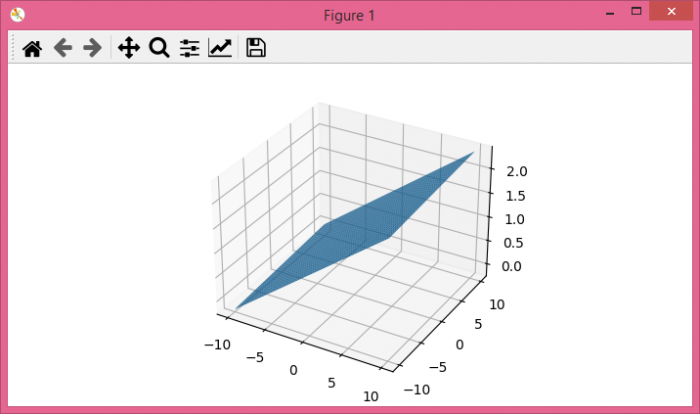# How to plot a plane using some mathematical equation in matplotlib?

To plot a plane using some mathematical equation in matplotlib, we can take the following steps.

## Steps

• Set the figure size and adjust the padding between and around the subplots.

• Create x and y data points using numpy.

• Using x and y, find the equation of the plane (eq).

• Create a new figure or activate an existing figure.

• Get the current axis with projection='3d'.

• Create a surface plot with x, y and eq data points.

• To display the figure, use Show() method.

## Example

import numpy as np
import matplotlib.pyplot as plt

plt.rcParams["figure.figsize"] = [7.00, 3.50]
plt.rcParams["figure.autolayout"] = True

x = np.linspace(-10, 10, 100)
y = np.linspace(-10, 10, 100)

x, y = np.meshgrid(x, y)
eq = 0.12 * x + 0.01 * y + 1.09

fig = plt.figure()

ax = fig.gca(projection='3d')

ax.plot_surface(x, y, eq)

plt.show()


## Output

It will produce the following output −